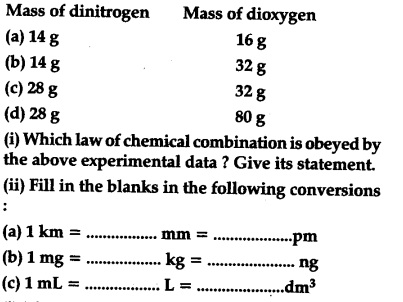# The following data are obtained when dinitrogen and dioxygen react together to form different compounds:

The following data are obtained when dinitrogen and dioxygen react together to form different compounds:The given data is in accordance with the law of multiple proportions, which states : When two elements combine to from two or more than two compounds, the weight of one of the two elements which combines with the fixed weight of the other, bears a simple ratio to one another,In the said question, if we fix the weight of dinitrogen at 14 g, then the weights of dioxygen which combines with the fixed weight (= 14 g) of dinitrogen will be 16,32,16,40 which are in the simple whole number ratio of 1:2:1:25 or 2:4:2: 5.
(ii) (a) 1 kg = \${{10}^{6}}\$ mm = \${{10}^{15}}\$ pm
(b) 1 mg = \${{10}^{-6}}\$ kg = \${{10}^{6}}\$ ng.